# Trigonometry Choosing Ratio Worksheet A The worksheet is

• Slides: 9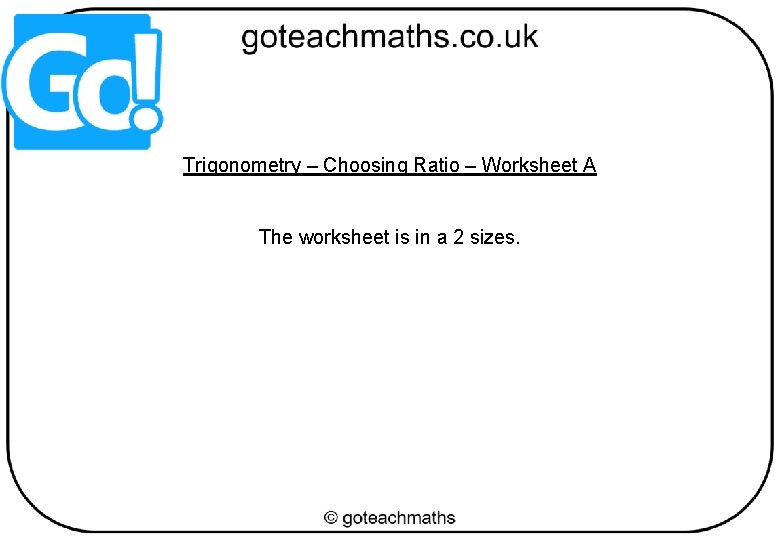Trigonometry – Choosing Ratio – Worksheet A The worksheet is in a 2 sizes.Printing To print handouts from slides Select the slide from the left. Then click: File > Print > ‘Print Current Slide’ To print multiple slides Click on a section title to highlight all those slides, or press ‘Ctrl’ at the same time as selecting slides to highlight more than one. Then click: File > Print > ‘Print Selection’ To print double-sided handouts Highlight both slides before using ‘Print Selection’. Choose ‘Print on Both Sides’ and ‘Flip on Short Edge’.Choosing a Trigonometric Ratio to Use SOH CAH or TOA For each triangle, decide whether you would use… = SOH p Hy Opp use ten po Opposite Hy ① Adjacent Adj = CAH Hy p Opp Hyp Adj ④ ③ O = TOA = SOH ⑤ 23° H 6 cm ⑥ A 18° ⑦ = TOA 10 cm O Adj ② 47° = CAH = SOH ⑧ ⑩ 7 c 32° 22 m = CAH = TOA 6 cm m m 81° 14 m ⑨ = SOH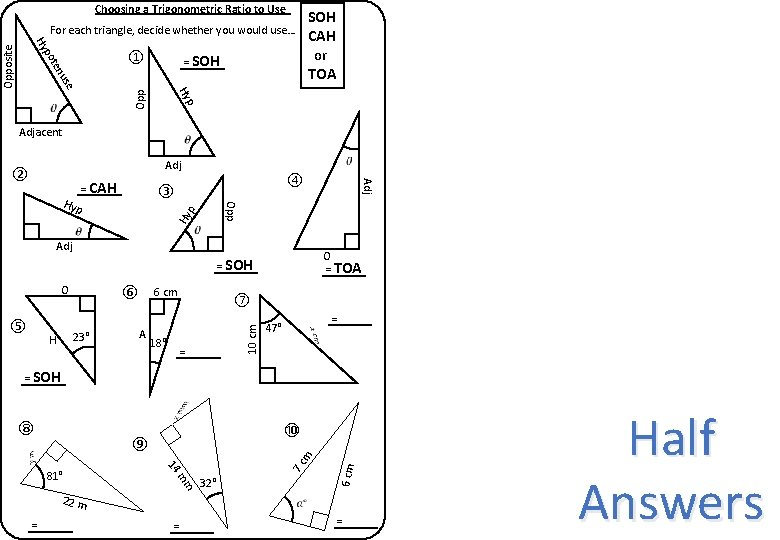Choosing a Trigonometric Ratio to Use SOH CAH or TOA For each triangle, decide whether you would use… = SOH p Hy Opp use ten po Opposite Hy ① Adjacent Adj = CAH Hy p Opp Hyp Adj ④ ③ O = TOA = SOH ⑤ 23° H 6 cm ⑥ A 18° ⑦ = TOA 10 cm O Adj ② 47° = CAH = SOH ⑧ ⑩ 7 c 32° 22 m = CAH = TOA 6 cm m m 81° 14 m ⑨ = SOH Half AnswersChoosing a Trigonometric Ratio to Use SOH CAH or TOA For each triangle, decide whether you would use… = SOH p Hy Opp use ten po Opposite Hy ① Adjacent Adj = CAH Hy p Opp Hyp Adj ④ ③ O = TOA = SOH ⑤ 23° H 6 cm ⑥ A 18° ⑦ = TOA 10 cm O Adj ② 47° = CAH = SOH ⑧ ⑩ 7 c 32° 22 m = CAH = TOA 6 cm m m 81° 14 m ⑨ = SOH Half Answers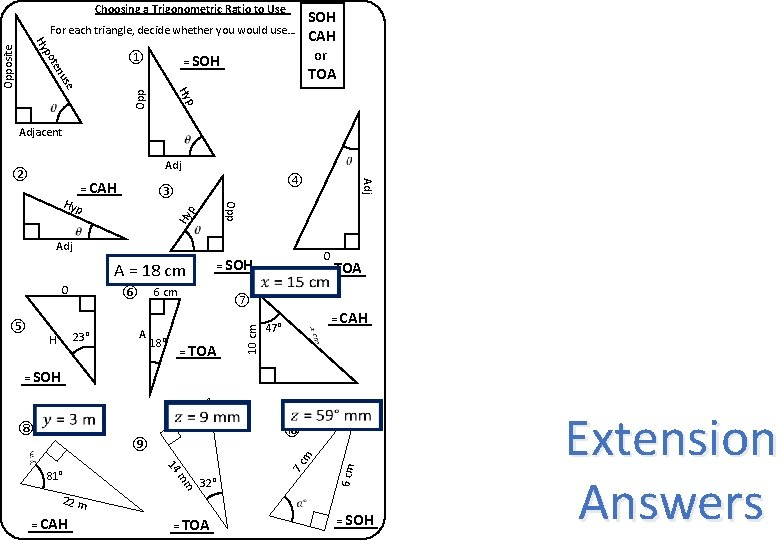Choosing a Trigonometric Ratio to Use SOH CAH or TOA For each triangle, decide whether you would use… = SOH p Hy Opp use ten po Adjacent Adj = CAH Hy p Opp Hyp Adj ④ ③ = SOH A = 18 cm O ⑤ 6 cm ⑥ 23° H Adj ② A 18° ⑦ = TOA O = TOA 10 cm Opposite Hy ① 47° = CAH = SOH ⑧ ⑩ 7 c 32° 22 m = CAH = TOA 6 cm m m 81° 14 m ⑨ = SOH Extension Answers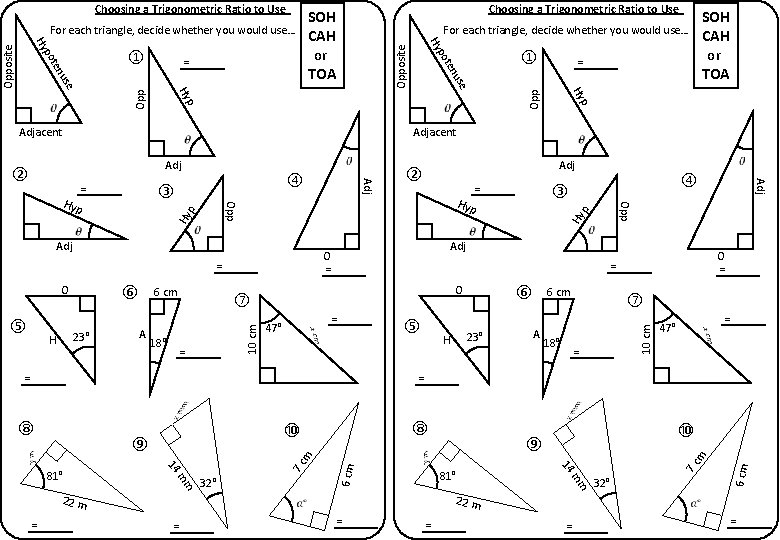O = TOA 10 cm = CAH ⑤ 23° H p Opp Hy A 18° ⑦ = TOA 47° = CAH = SOH ⑧ ⑧ ⑩ ⑩ ⑨ = CAH = TOA m 7 c 6 cm = SOH m 22 m 81° m 32° 14 m m 14 81° 7 c m ⑨ O = TOA 32° 22 m = CAH = TOA 6 cm Opposite = SOH 6 cm ⑥ 10 cm O 47° = SOH ⑦ = TOA Adj ④ ③ Opp 18° p A Hy Adj 6 cm ⑥ 23° H = CAH Hyp = SOH ⑤ Adj ② O Opp Adj ④ ③ Hyp = SOH Adjacent Adj = CAH use p ② ten Hy Adjacent ① po use ten po Hy Hy = SOH CAH or TOA For each triangle, decide whether you would use… Opposite For each triangle, decide whether you would use… ① Choosing a Trigonometric Ratio to Use SOH CAH or TOA Opp Choosing a Trigonometric Ratio to Use = SOH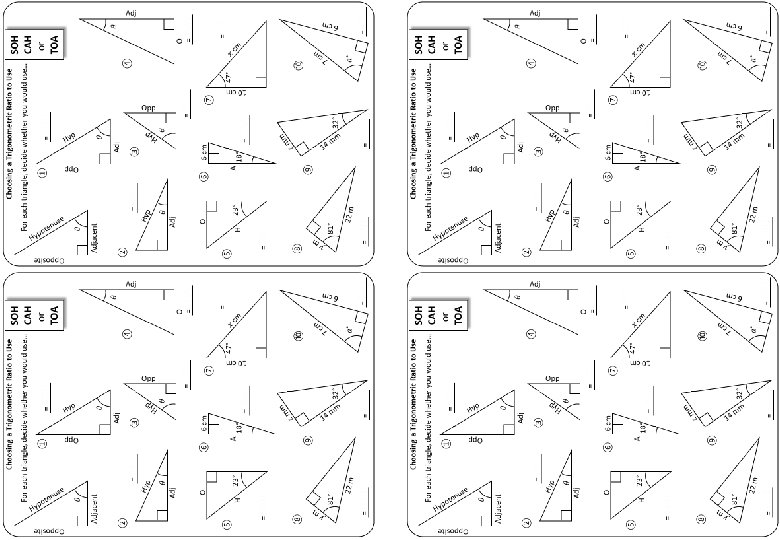Questions? Comments? Suggestions? …or have you found a mistake!? Any feedback would be appreciated . Please feel free to email: [email protected] co. uk